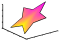fill3

Filled 3-D polygonsSyntax

fill3(X,Y,Z,C)
fill3(X,Y,Z,ColorSpec)
fill3(X1,Y1,Z1,C1,X2,Y2,Z2,C2,...)
fill3(...,'PropertyName',PropertyValue)
fill3(ax,...)
h = fill3(...)

Description

fill3(X,Y,Z,C) fills three-dimensional polygons. X, Y, and Z triplets specify the polygon vertices. If X, Y, or Z is a matrix, fill3 creates n polygons, where n is the number of columns in the matrix. fill3 closes the polygons by connecting the last vertex to the first when necessary. The values in X, Y, and Z can be numeric, datetime, duration, or categorical values.

C specifies color, where C is a vector or matrix of indices into the current colormap. If C is a row vector, length(C) must equal size(X,2) and size(Y,2); if C is a column vector, length(C) must equal size(X,1) and size(Y,1).

fill3(X,Y,Z,ColorSpec) fills the polygons with the color specified by ColorSpec, which can be one of the following values:

• A color name or a short name: 'red' (or 'r'), 'green' (or 'g'), 'blue' (or 'b'), 'cyan' (or 'c'), 'magenta' (or 'm'), 'yellow' (or 'y'), 'black' (or 'k'), 'white' (or 'w').

• An RGB triplet specified as a 1-by-3 vector whose elements are the intensities of the red, green, and blue components of a color. The intensities must be in the range [0, 1]. For example, [0.7 0.8 1] specifies light blue.

fill3(X1,Y1,Z1,C1,X2,Y2,Z2,C2,...) specifies multiple filled three-dimensional areas.

fill3(...,'PropertyName',PropertyValue) allows you to set values for specific patch properties.

fill3(ax,...) creates the polygons in the axes specified by ax instead of in the current axes (gca). The option ax can precede any of the input argument combinations in the previous syntaxes.

h = fill3(...) returns a vector of patch objects.

Examples

collapse all

Create four triangles with interpolated colors.

X = [0 1 1 2; 1 1 2 2; 0 0 1 1];
Y = [1 1 1 1; 1 0 1 0; 0 0 0 0];
Z = [1 1 1 1; 1 0 1 0; 0 0 0 0];
C = [0.5000 1.0000 1.0000 0.5000;
1.0000 0.5000 0.5000 0.1667;
0.3330 0.3330 0.5000 0.5000];

figure
fill3(X,Y,Z,C)Algorithms

If X, Y, and Z are matrices of the same size, fill3 forms a vertex from the corresponding elements of X, Y, and Z (all from the same matrix location), and creates one polygon from the data in each column.

If X, Y, or Z is a matrix, fill3 replicates any column vector argument to produce matrices of the required size.

If you specify color using the ColorSpec argument, fill3 generates flat-shaded polygons and sets the patch object FaceColor property to an RGB triplet.

If you specify color using C, fill3 scales the elements of CLim by the axes property CLim, which specifies the color axis scaling parameters, before indexing the current colormap.

If C is a row vector, fill3 generates flat-shaded polygons and sets the FaceColor property of the patch objects to 'flat'. Each element becomes the CData property value for the respective patch object.

If C is a column vector or a matrix, fill3 generates polygons with interpolated colors and sets the patch object FaceColor property to 'interp'. fill3 uses a linear interpolation of the vertex colormap indices when generating polygons with interpolated colors. The elements in one column become the CData property value for the respective patch object. If C is a column vector, fill3 replicates the column vector to produce the required sized matrix.

Compatibility Considerations

expand all

Behavior changed in R2021a